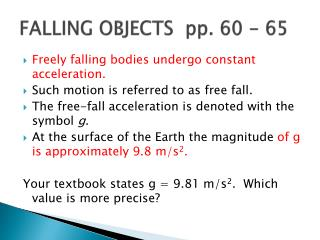# FALLING OBJECTS pp. 60 - 65 - PowerPoint PPT PresentationDownload PresentationFALLING OBJECTS pp. 60 - 65

FALLING OBJECTS pp. 60 - 65Download Presentation## FALLING OBJECTS pp. 60 - 65

- - - - - - - - - - - - - - - - - - - - - - - - - - - E N D - - - - - - - - - - - - - - - - - - - - - - - - - - -
##### Presentation Transcript

1. FALLING OBJECTS pp. 60 - 65 • Freely falling bodies undergo constant acceleration. • Such motion is referred to as free fall. • The free-fall acceleration is denoted with the symbol g. • At the surface of the Earth the magnitude of g is approximately9.8 m/s2. Your textbook states g = 9.81 m/s2. Which value is more precise?

2. Falling objects • In our usual choice of coordinates, the downward direction is negative. Thus, the acceleration of objects in free fall near the surface of the earth is a = g = -9.8 m/s2. • Gravity acts in a downward direction; therefore, you may tend to consider g as positive. This works as long as you are consistent with your sign convention. • Freely falling objects always have the same downward acceleration.

3. Falling Objects Jason hits a volleyball so that it moves with an initial velocity of 6.0 m/s straight upward. If the volleyball starts from 2.0 m above the floor, how long will it be in the air before it strikes the floor? Assume that Jason is the last player to touch the ball before it hits the floor?

4. Problem p.63 6.0 m/s 2.0 m

5. Remember the kinematic equations:

6. Solving the problem: Both t and vf are unknown Solve for vf using an acceleration equation Solve for t using the other acceleration equation • Vf = -8.7 m/s • ∆t = 1.5 sec

7. Is your answer reasonable? p. 64 • Because the velocity will decrease by 9.81 m/s in 1 s and because vi is only 6.0 m/s, it will take a little less than 1 s for the ball to reach its maximum height. Once the ball is at its maximum height, it will take less than 1 sec to fall to its original position and a little additional time to fall the final 2.0 m to the floor. Therefore, a total time of between 1.0 s and 2.0 s is reasonable.

8. Reaction Time Lab • Let’s discuss the reaction time lab done in the last class. • Always comment on any negative values.

9. Practice problemsPlease use the white boards. Write final analysis in your notebook. • 1) A robot probe drops a camera off the rim of a 239 m high cliff on Mars, where the free-fall acceleration is -3.7m/s2. a) Find the velocity with which the camera hits the ground. (-4.2 m/s) b) Find the time required for it to hit the ground. (11 sec)

10. Problems (cont.) • 1) A robot probe drops a camera off the rim of a 239 m high cliff on Mars, where the free-fall acceleration is -3.7m/s2. a) Find the velocity with which the camera hits the ground. (-4.2 m/s) b) Find the time required for it to hit the ground. (11 sec) • 2) A flower pot falls from a windowsill 25.0 m above the sidewalk. • How fast is the flowerpot moving when it strikes the ground? (22.1 m/s) • How much time does a passerby on the sidewalk below have to move out of the way before the flowerpot hits the ground? (2.25 sec)

11. Problems (cont.) • 2) A flower pot falls from a windowsill 25.0 m above the sidewalk. • How fast is the flowerpot moving when it strikes the ground? (22.1 m/s) • How much time does a passerby on the sidewalk below have to move out of the way before the flowerpot hits the ground? (2.25 sec) • 3. A tennis ball is thrown vertically upward with an initial velocity of +8.0 m/s. • What will the ball’s speed be when it returns to it’s starting point? (8 m/s) • How long will it take the ball to reach its starting point? (1.63 sec)